# ggplot2作图详解3：映射（mapping）

## 1 映射的类型

ggplot2按照图形属性提供了以下可用映射类型：

• 颜色类型映射：包括 color（颜色或边框颜色）、fill（填充颜色）和 alpha（透明度）
• 形状类型映射：包括 linetype（线型）、size（点的大小或线的宽度）和 shape（形状）
• 位置类型映射：包括 x, y, xmin, xmax, ymin, ymax, xend, yend
• 特殊类型：包括两类，一类是指定数据分组和顺序的映射group和order，另一类是字符串映射。

## 2 颜色和形状类型映射

### 2.1 映射的过程

```library(ggplot2)
set.seed(100)
d.sub <- diamonds[sample(nrow(diamonds), 500), ]
##       carat       cut color clarity depth table price    x    y    z
## 16601  1.01 Very Good     D     SI1  62.1    59  6630 6.37 6.41 3.97
## 13899  0.90     Ideal     D     SI1  62.4    55  5656 6.15 6.19 3.85
## 29792  0.30     Ideal     D     SI1  61.6    56   709 4.34 4.30 2.66
## 3042   0.30 Very Good     G     VS1  62.0    60   565 4.27 4.31 2.66```

`p  <- ggplot(data=d.sub, aes(x=carat, y=price))`

```theme_set(theme_bw())
p  + geom_point()```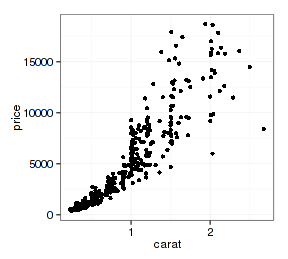`p  + geom_point() + aes(color=color) + theme(legend.position=c(2,2))`

ggplot2映射的过程可以用plot函数作图步骤进行分解，它包含三方面的操作（不包括图形页面设置）：

• 数据分组
• 设定颜色标尺
• 按颜色标尺指定每组数据的颜色
```# 设定颜色标尺
levs  <- levels(d.sub\$color)
cl  <-  rainbow(length(levs))
# 页面设置
par(mar=c(3,3,0.5,0.5), mgp=c(1.5, 0.5, 0), bg="white")
plot(d.sub\$carat, d.sub\$price, type='n', xlab="carat", ylab="price")
i  <- 1
for(lev in levs){
# 数据分组
datax  <- d.sub[d.sub\$color==lev, ]
# 作图并指定数据点颜色
points(datax\$carat, datax\$price, pch=20, col=cl[i])
i  <- i + 1
}```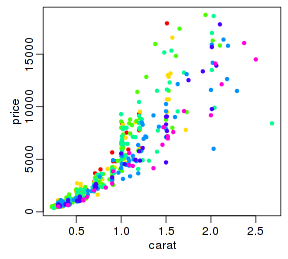`p  + geom_point() + aes(color=color, shape=cut) + theme(legend.position=c(2,2))`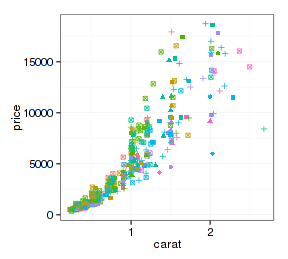### 2.2 映射的标尺

• 离散型（或枚举型）标尺。罗列出所有数据分类并将其与美学属性一一对应，处理过程包含数据分类，如上例。
• 连续型（或区间型）标尺。看下面例子：
`p  + geom_point() + aes(size= x*y*z ) + theme(legend.position=c(2,2))`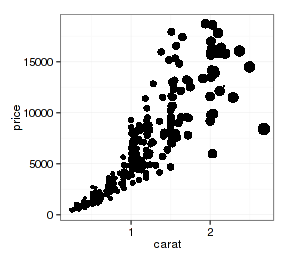```cex <- d.sub[,"x"]*d.sub[,"y"]*d.sub[,"z"]
cex  <- cex/max(cex)*4
par(mar=c(3,3,0.5,0.5), mgp=c(1.5, 0.5, 0), bg="white")
plot(d.sub\$carat, d.sub\$price, type='n', xlab="carat", ylab="price")
points(d.sub[, "carat"], d.sub[, "price"], pch=20, cex=cex)```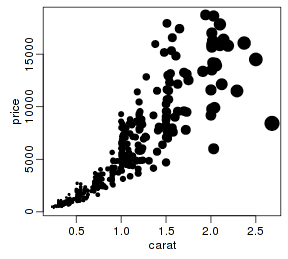ggplot2对映射应用的标尺可以修改，ggplot提供了一大批 scale_xxxxxxxx 类型的函数，比如 scale_color_xxxx 类型函数用户修改颜色标尺，scale_shape_xxxx 修改形状，scale_linetype_xxxx 修改线型等。按照数据的类型，这些函数还有4种基本类型：

• continuous：连续型
• discrete：离散型
• identity：和数据取值相同
• manual：手工指定

```cls  <- terrain.colors(length(levels(d.sub\$color)))
p  + geom_point() + aes(color=color) + scale_color_manual(values=cls)```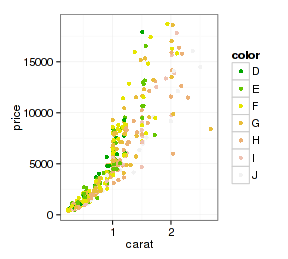### 2.4 图形颜色和形状的非映射设置

```p  + geom_boxplot(aes(x=cut, fill=cut)) +
scale_fill_manual(values=rep("cyan", length(levels(d.sub\$cut))))
p  + geom_boxplot(aes(x=cut), fill="cyan")```

```qplot(x=cut, y=price, data=d.sub, geom="boxplot", fill="cyan")
qplot(x=cut, y=price, data=d.sub, geom="boxplot", fill=I("cyan"))```

“I”函数表示设为固定值，如果不是在qplot函数中可以不用它。

```p + geom_point(data=diamonds, aes(alpha=carat/100))
p + geom_point(data=diamonds, alpha=0.05)```

## 3 位置类型映射

x和y映射的用法很明确，就不再罗嗦了。xmin, xmax, ymin, ymax, xend, yend这几种映射属于特殊类型，H.W.在ggplot2的说明档里面写得很清楚（赞，H.W.不仅是写R软件的高手，还是普及教育的牛人），下面就照搬他的例子简单说明一下用法。

### 3.1 ymin/ymax映射的用法

```dmod <- lm(price ~ cut, data = diamonds)
cuts <- data.frame(cut = unique(diamonds\$cut), predict(dmod, data.frame(cut =
unique(diamonds\$cut)), se = TRUE)[c 1=""se.fit")" language="("fit","][/c])
cuts
##         cut  fit se.fit
## 1     Ideal 3458  27.00
## 3      Good 3929  56.59
## 4 Very Good 3982  36.06
## 5      Fair 4359  98.79```

```se <- ggplot(cuts, aes(x = cut, y = fit, ymin = fit - se.fit, ymax = fit + se.fit, colour = cut))
se + geom_pointrange()```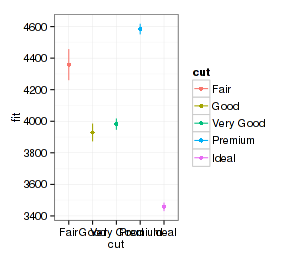```se + geom_point() + geom_errorbar(width=0.2)
se + geom_bar(stat="identity", aes(fill=cut)) + geom_errorbar(width=0.2)```

min/ymax可以用来改变几何类型的坐标轴范围，但 这不是标准用法 ，最简单的是用ylim函数：

```se + geom_point(aes(ymin = 3000, ymax = 4800)) + geom_errorbar(width=0.2)
se + geom_point() + geom_errorbar(width=0.2) + ylim(3000,4800)
# 或者用： scale_y_continuous(limits=c(3000,5000))```

```se + geom_bar(stat="identity", aes(fill=cut)) +
geom_errorbar(width=0.2) + coord_cartesian(ylim=c(3000,4800))```

```px <- ggplot(mtcars, aes(wt, mpg)) + geom_point()
px + annotate("rect", xmin = 2, xmax = 3.5, ymin = 2, ymax = 25, fill = "dark grey", alpha = .5)
library(grid)
px + geom_segment(aes(x = 5, y = 30, xend = 3.5, yend = 25), arrow = arrow(length = unit(0.5, "cm")))```

## 4 特殊类型映射

```ggplot(d.sub, aes(x=carat, y=price, color=cut)) +
geom_point() + geom_smooth(method="lm", se=FALSE)```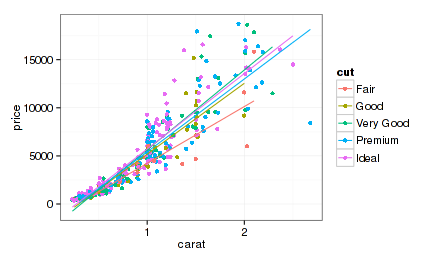```ggplot(d.sub, aes(x=carat, y=price, color=cut)) +
geom_point() + geom_smooth(aes(group=1), method="lm", se=FALSE)
ggplot(d.sub, aes(x=carat, y=price, color=cut)) + geom_point() +
geom_smooth(aes(group=color, linetype=color), method="lm", se=FALSE, color="black")```

```se + geom_bar(stat="identity", aes(fill=cut)) + geom_errorbar(width=0.2) +
coord_cartesian(ylim=c(3000,4800)) + geom_line(aes(group=1), color="black")```

order映射用于改变数据类型的排序。注意是在图层中的排列顺序而不是图例的先后顺序。看下面图形颜色的差别：

```p + geom_point(aes(color=cut, order=cut))
library(plyr) # 使用desc函数
p + geom_point(aes(color=cut, order=desc(cut)))```

aes_string太过另类，不说了。

## 5 SessionInfo

```sessionInfo()
## R version 3.1.0 (2014-04-10)
## Platform: x86_64-pc-linux-gnu (64-bit)
##
## locale:
##   LC_CTYPE=zh_CN.UTF-8       LC_NUMERIC=C
##   LC_TIME=zh_CN.UTF-8        LC_COLLATE=zh_CN.UTF-8
##   LC_MONETARY=zh_CN.UTF-8    LC_MESSAGES=zh_CN.UTF-8
##   LC_PAPER=zh_CN.UTF-8       LC_NAME=C
##  LC_MEASUREMENT=zh_CN.UTF-8 LC_IDENTIFICATION=C
##
## attached base packages:
##  grid      tcltk     stats     graphics  grDevices utils     datasets
##  methods   base
##
## other attached packages:
##  plyr_1.8.1      ggplot2_0.9.3.1 zblog_0.1.0     knitr_1.5
##
## loaded via a namespace (and not attached):
##   colorspace_1.2-4 digest_0.6.4     evaluate_0.5.3   formatR_0.10
##   gtable_0.1.2     highr_0.3        labeling_0.2     MASS_7.3-31
##   munsell_0.4.2    proto_0.3-10     Rcpp_0.11.1      reshape2_1.2.2
##  scales_0.2.4     stringr_0.6.2    tools_3.1.0```

• 本文由 发表
• 转载请务必保留本文链接：https://www.plob.org/article/7624.html